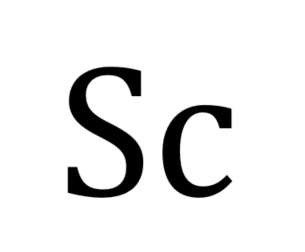f
Neutrium

# Sherwood Number

Index of Dimensionless Numbers

Dimensionless numbers play an important role in analysing fluid dynamics and heat and mass transfer problems. They provide a method by which complex phenomena can be characterised, often by way of a simple, single number comparison. This article provides a summary of dimensionless numbers and the formulae used to calculate them.

Dimensionless Numbers and Dimensional Analysis

Dimensionless numbers play an important role in analysing fluid dynamics and heat and mass transfer problems. They provide a method by which complex phenomena can be characterised, often by way of a simple, single number comparison. This article provides a brief overview of the derivation and use of dimensionless numbers.

Sherwood NumberThe Sherwood number is a dimensionless number that represents the ratio of convective mass transfer to the rate of diffusive mass transport and is used in the analysis of mass transfer systems such as liquid-liquid extraction. This article describes the Sherwood number and typical formulations.

Schmidt NumberThe Schmidt number is a dimensionless number that describes the ratio of momentum diffusivity to mass diffusivity that is commonly used in analysis of mass transfer systems. This article describes the Schmidt Number and typical formulations.